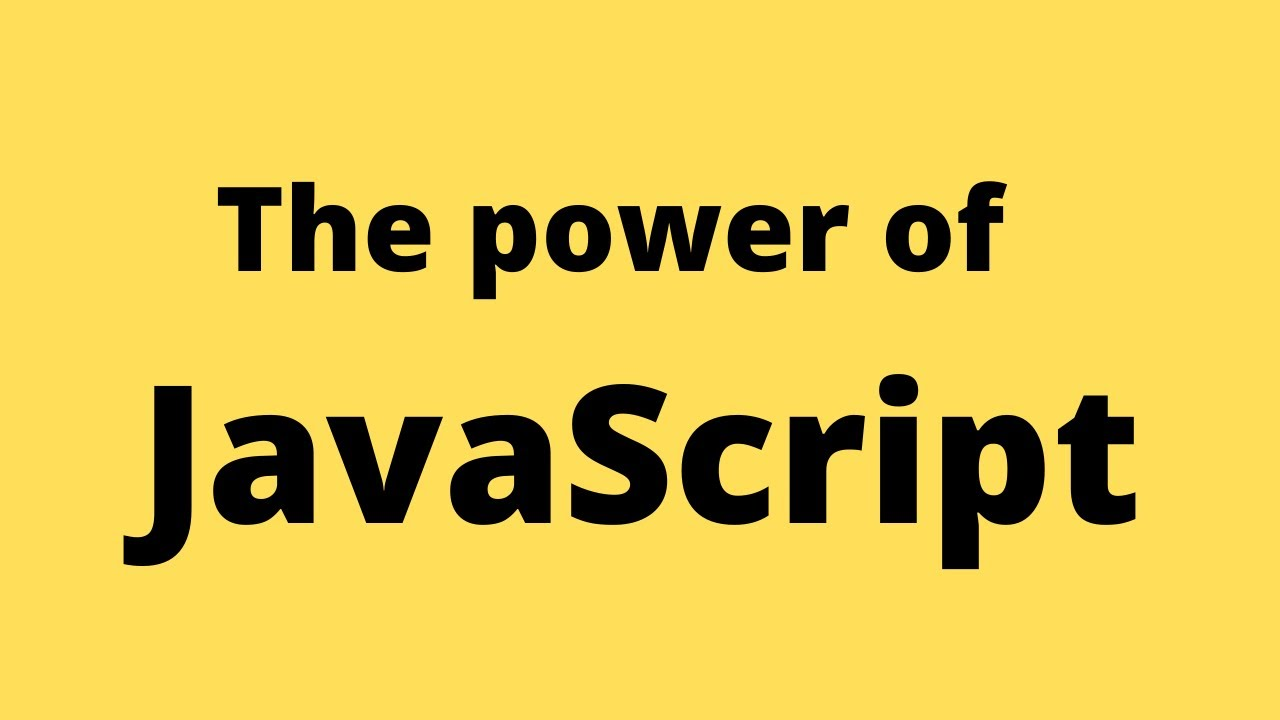1594048641

# JavaScript tutorial - array.prototype.flat()

Javascript Array flat() is an inbuilt method that creates a new array with all sub-array elements concatenated into it recursively up to the specified depth. It returns a new array with the sub-array elements concatenated into it.This tutorial covers array.prototype.flat() method. A multiple examples have been provided.

## Javascript Array Flat Example

As its name suggests, the Array flat() method available on an Array prototype returns the new array that’s the flattened version of an array it was called on. If we do not provide arguments passed-in, then the depth of 1 is assumed.

The syntax of the Javascript Array flat() method is the following.

``````let newArray = arr.flat([depth]);
``````

The depth level is specifying how deep a nested array structure should be flattened. Defaults to 1. It is an optional parameter.

Let’s see the following example.

``````// app.js

const arr = [['krunal', 'ankit'], [21, 74]];

console.log(arr.flat());
``````

See the output.In the above example, by default, the depth level is 1. Let’s see the example where depth level is more than 1.

``````// app.js

const arr = [['krunal', 'ankit'], [21, 74], ['appdividend', ['Laravel', 'Angular']]];

console.log(arr.flat(2));
``````

#javascript #array #prototype #flat #programming

## Buddha Community1624388400

## JavaScript Arrays Tutorial. DO NOT MISS!!!

Learn JavaScript Arrays

📺 The video in this post was made by Programming with Mosh
The origin of the article: https://www.youtube.com/watch?v=oigfaZ5ApsM&list=PLTjRvDozrdlxEIuOBZkMAK5uiqp8rHUax&index=4
🔥 If you’re a beginner. I believe the article below will be useful to you ☞ What You Should Know Before Investing in Cryptocurrency - For Beginner
⭐ ⭐ ⭐The project is of interest to the community. Join to Get free ‘GEEK coin’ (GEEKCASH coin)!
Thanks for visiting and watching! Please don’t forget to leave a like, comment and share!

#arrays #javascript #javascript arrays #javascript arrays tutorial1594048641

## JavaScript tutorial - array.prototype.flat()

Javascript Array flat() is an inbuilt method that creates a new array with all sub-array elements concatenated into it recursively up to the specified depth. It returns a new array with the sub-array elements concatenated into it.This tutorial covers array.prototype.flat() method. A multiple examples have been provided.

## Javascript Array Flat Example

As its name suggests, the Array flat() method available on an Array prototype returns the new array that’s the flattened version of an array it was called on. If we do not provide arguments passed-in, then the depth of 1 is assumed.

The syntax of the Javascript Array flat() method is the following.

``````let newArray = arr.flat([depth]);
``````

The depth level is specifying how deep a nested array structure should be flattened. Defaults to 1. It is an optional parameter.

Let’s see the following example.

``````// app.js

const arr = [['krunal', 'ankit'], [21, 74]];

console.log(arr.flat());
``````

See the output.In the above example, by default, the depth level is 1. Let’s see the example where depth level is more than 1.

``````// app.js

const arr = [['krunal', 'ankit'], [21, 74], ['appdividend', ['Laravel', 'Angular']]];

console.log(arr.flat(2));
``````

#javascript #array #prototype #flat #programming1599726120

## Javascript Array Flat Example | Array.prototype.flat()

Javascript Array flat() is an inbuilt method that creates a new array with all sub-array elements concatenated into it recursively up to the specified depth. It returns a new array with the sub-array elements concatenated into it.

### Javascript Array Flat Example

As its name suggests, the Array flat() method available on an Array prototype returns the new array that’s the flattened version of an array it was called on. If we do not provide arguments passed-in, then the depth of 1 is assumed.

The syntax of the Javascript Array flat() method is the following.

``````let newArray = arr.flat([depth]);
``````

The depth level is specifying how deep a nested array structure should be flattened. Defaults to 1. It is an optional parameter.

#javascript #flat #array.prototype.flat #js1600410360

## Javascript Array From Example | Array.prototype.from()

Javascript array from() is an inbuilt function that creates a new, shallow-copied array instance from an array-like object or iterable object.

The Array .from() lets you develop Arrays from the array-like objects (objects with a length property and indexed items) or  iterable objects ( objects where you can get its items, such as Map and  Set).

The Array from() function was introduced in ECMAScript 2015.

### Javascript Array From Example

Array.from() method in Javascript is used to creates a new  array instance from a given array. If you pass a  string to the Array.from() function, then, in that case, every alphabet of the string is converted to an element of the new array instance. In the case of integer values, a new array instance simply takes the elements of the given Array.

The syntax of the Array.from() method is the following.

#### Syntax

``````Array.from(arrayLike[, mapFn[, thisArg]])
``````

#javascript #ecmascript #javascript array from #array.prototype.from1600506180

## Javascript Array Shift Example | Array.prototype.shift()

Javascript array shift() is an inbuilt function that removes the first item from an array and returns that deleted item. The shift() method changes the length of the array on which we are calling the shift() method. Javascript Array Shift method is not  pure function as it directly modifies the  array.

### Javascript Array Shift Example

Javascript shift() method removes the item at the zeroeth index and shifts the values at consecutive indexes down, then returns that removed value.

If we want to remove the last item of an array, use the  Javascript pop() method.

If the length property is 0,  undefined is returned.

The syntax for shift() method is the following.

``````array.shift()
``````

An array element can be a  string, a number, an  array, a boolean, or any other  object types that are allowed in the Javascript array. Let us take a simple example.

#javascript #array.prototype.shift #javascript pop #javascript shift